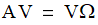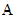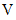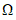# NMath User's Guide

Chapter 23. EigenValue Problems (.NET, C#, CSharp, VB, Visual Basic, F#)

NMath includes classes for solving symmetric, Hermitian, and nonsymmetric eigenvalue problems. The classical eigenvalue problem is defined as the solution to:for a matrix, eigenvectors, and the diagonal matrix of eigenvalues. NMath also provides eigenvalue server classes that construct instances of the eigenvalue classes, allowing you greater control over how the eigenvalue decomposition is performed.

For example, class DoubleSymEigDecomp computes the eigenvalues and eigenvectors of a DoubleSymmetricMatrix. By default, this class computes both eigenvalues and eigenvectors. For more control, the associated decomposition server class, DoubleSymEigDecompServer, can be configured to compute eigenvalues only, or both eigenvalues and eigenvectors. In addition, the server can be configured to compute only the eigenvalues in a given range. A tolerance for the convergence of the algorithm may also be specified.

This chapter describes the NMath eigenvalue and eigenvalue server classes, and how to construct and use them.

Top

Top